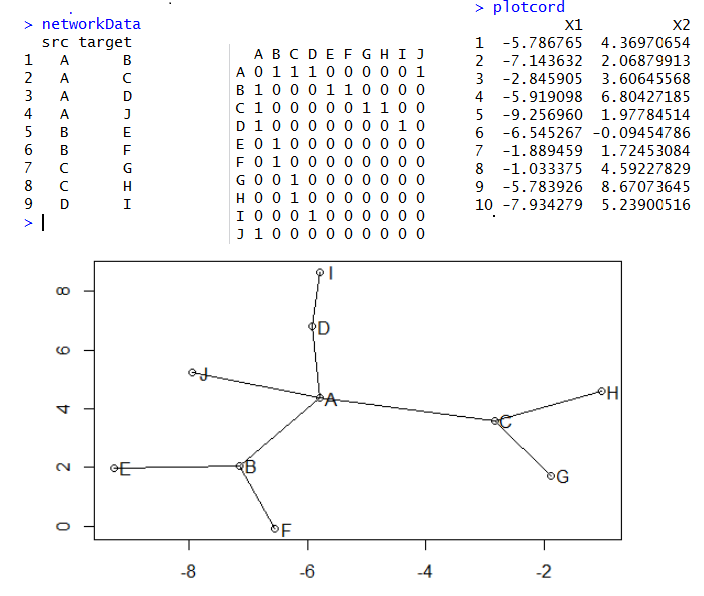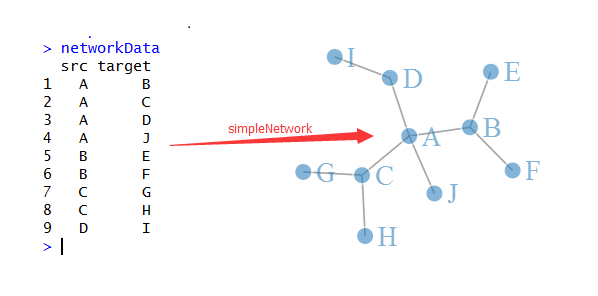cytoscape五步曲之二：在cytoscape里面生成网络图

http://wiki.cytoscape.org/Cytoscape_User_Manual/Network_Formats 当然，其实木有意义！因为我们不可能拿到cytoscape的输入文件（cys格式的），除非是你朋友传给你的。我们肯定是根据txt.csv等分割的文本文件来做网络图。

14

R语言画网络图三部曲之sna

plot(plotcord)
text(x=plotcord\$X1+0.2,y=plotcord\$X2,labels = LETTERS[1:10])
for (i in 1:10){
for (j in 1:10){
if(tmp[i,j]) lines(plotcord[c language="(i,j),1"][/c],plotcord[c language="(i,j),2"][/c])
}
}net=network(150, directed=FALSE, density=0.03)
m <- as.matrix.network.adjacency(net) # get sociomatrix
# get coordinates from Fruchterman and Reingold's force-directed placement algorithm.
plotcord <- data.frame(gplot.layout.fruchtermanreingold(m, NULL))
# or get it them from Kamada-Kawai's algorithm:
colnames(plotcord) = c("X1","X2")  ###所有点的坐标，共150个点
edglist <- as.matrix.network.edgelist(net) ##所有点之间的关系-edge ##共335条线
edges <- data.frame(plotcord[edglist[,1],], plotcord[edglist[,2],]) ##两点之间的连线的具体坐标，335条线的起始终止点点坐标

library(network)
library(ggplot2)
library(sna)
library(ergm)

14

R语言画网络图三部曲之networkD3

# Plot
library(networkD3)
simpleNetwork(networkData,fontSize=25)sankeyNetwork
sankeyNetwork